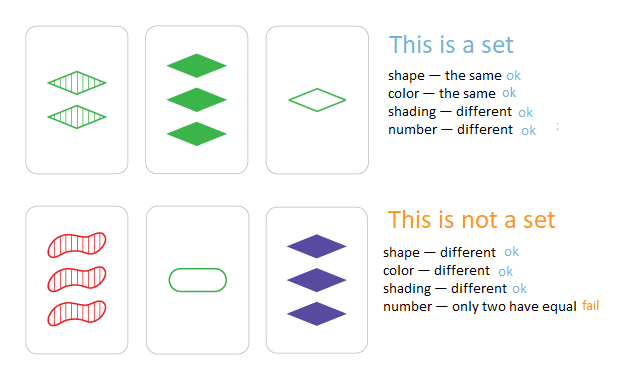# Hyperset（排序＋二分）

Bees Alice and Alesya gave beekeeper Polina famous card game “Set” as a Christmas present. The deck consists of cards that vary in four features across three options for each kind of feature: number of shapes, shape, shading, and color. In this game, some combinations of three cards are said to make up a set. For every feature — color, number, shape, and shading — the three cards must display that feature as either all the same, or pairwise different. The picture below shows how sets look.Polina came up with a new game called “Hyperset”. In her game, there are nn cards with kk features, each feature has three possible values: “S”, “E”, or “T”. The original “Set” game can be viewed as “Hyperset” with k=4k=4.

Similarly to the original game, three cards form a set, if all features are the same for all cards or are pairwise different. The goal of the game is to compute the number of ways to choose three cards that form a set.

Unfortunately, winter holidays have come to an end, and it’s time for Polina to go to school. Help Polina find the number of sets among the cards lying on the table.

Input
The first line of each test contains two integers nn and kk (1≤n≤15001≤n≤1500, 1≤k≤301≤k≤30) — number of cards and number of features.

Each of the following nn lines contains a card description: a string consisting of kk letters “S”, “E”, “T”. The ii-th character of this string decribes the ii-th feature of that card. All cards are distinct.

Output
Output a single integer — the number of ways to choose three cards that form a set.

Examples
Input
3 3
SET
ETS
TSE
Output
1
Input
3 4
SETE
ETSE
TSES
Output
0
Input
5 4
SETT
TEST
EEET
ESTE
STES
Output
2
Note
In the third example test, these two triples of cards are sets:

“SETT”, “TEST”, “EEET”
“TEST”, “ESTE”, “STES”

#include<cstdio>
#include<cstring>
#include<iostream>
#include<algorithm>
#include<map>
using namespace std;

const int maxx=1e4+100;
string s[maxx];
int n,m;

inline string fcs(string a,string b)
{
string c="";
int x,y;
for(int i=0;i<m;i++)
{
x=a[i]-'0';
y=b[i]-'0';
if((x^y)==0) c+=(x+'0');
else c+=((x^y)+'0');
}
return c;
}
int main()
{
scanf("%d%d",&n,&m);
int cnt=0;
for(int i=0;i<n;i++)
{
cin>>s[i];
string a="";
for(int j=0;j<m;j++)
{
if(s[i][j]=='S') a+='1';
else if(s[i][j]=='E') a+='2';
else a+='3';
}
s[i]=a;
}
int ans=0;
sort(s,s+n);
//for(int i=0;i<n;i++) cout<<s[i]<<endl;
for(int i=0;i<n;i++)
{
for(int j=i+1;j<n;j++)
{
string t=fcs(s[i],s[j]);
int l=0,r=n-1;
while(l<=r)
{
int mid=l+r>>1;
if(s[mid]==t)
{
ans++;
break;
}
else if(s[mid]>t) r=mid-1;
else l=mid+1;
}
}
}
printf("%d\n",ans/3);
return 0;
}


©️2019 CSDN 皮肤主题: 大白 设计师: CSDN官方博客